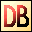DelphiBasicsPiFunction The mathematical constant System unit
 function Pi : Extended;
Description
The Pi function returns a floating point value giving a useful approximation of the value of Pi.

The circumference of a circle Pi * Diameter.
Related commands
 Cos The Cosine of a number Extended The floating point type with the highest capacity and precision Sin The Sine of a number Tan The Tangent of a numberDownload this web site as a Windows program.